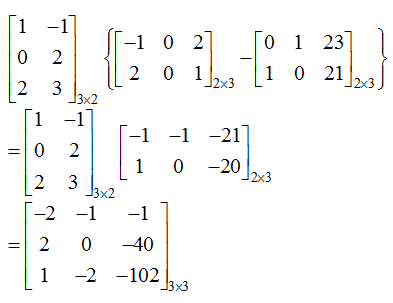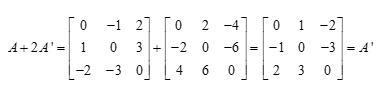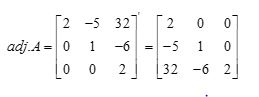# Topic: Matrices (Test 1)

Topic: Matrices
Q.1
If A and B are symmetric matrices of the same order, then
A. AB – BA is a symmetric matrix
B. A – B is a skew-symmetric matrix
C. AB + BA is a symmetric matrix
D. AB is symmetric matrix
Explaination / Solution:

If A and B are symmetric matrices of the same order, then AB + BA is always a symmetric matrix.

Workspace
Report
Q.2
If  then A is
A. a nilpotent matrix
B. an invertible matrix
C. an idempotent matrix
D. none of these
Explaination / Solution:

A square matrix A for which, where n is a positive integer, is called a Nilpotent matrix.

The determinant and trace of the matrix is always Zero for a Nilpotent Matrix.

For the given matrix "A", determinant (A)=0 and trace(A)=0.

Workspace
Report
Q.3
A square matrix A is called idempotent if
A. A2=O
B. 2A=I
C. A2=A
D. A2=I
Explaination / Solution:

If the product of any square matrix with itself is the matrix itself , then the matrix is called Idempotent.

Workspace
Report
Q.4
Let A be any  matrix, then  can be found only when
A. none of these.
B. m = n
C. m > n
D. m < n
Explaination / Solution:

The product of any matrix with itself can be found only when it is a square matrix.i.e. m=n.

Workspace
Report
Q.5
If where  then A is equal to
A.B.C.D.Explaination / Solution:

If where then
Workspace
Report
Q.6
f A and B are two matrices such that A + B and AB are both defined, then
A. A and B can be any matrices
B. A, B are square matrices not necessarily of same order
C. number of columns of A = number of ros of B.
D. A, B are square matrices of same order
Explaination / Solution:

If A and B are square matrices of same order , both operations A + B and AB are well defined.

Workspace
Report
Q.7A. 3 × 3
B. 2 × 2
C. 2 × 3
D. 3 × 2
Explaination / Solution:Workspace
Report
Q.8
Each diagonal element of a skew-symmetric matrix is
A. Zero
B. negative.
C. Positive
D. non-real
Explaination / Solution:

The diagonal elements of a skew-symmetric is zero.

Workspace
Report
Q.9A. At
B. A
C. At
D. 2A2
Explaination / Solution:Workspace
Report
Q.10A.B.C.D.